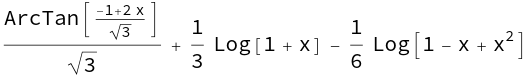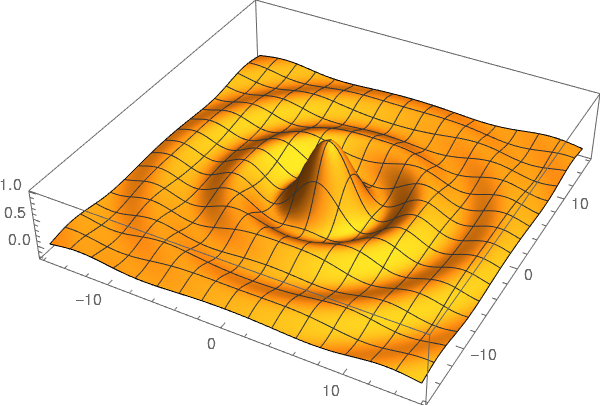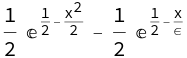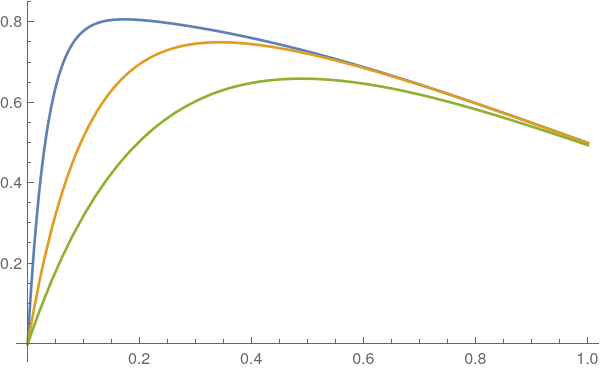# Example of Wolfram Language in Jupyter¶

This is a example of the use of the Wolfram Language inside Jupyter notebook. This provides an alternative to Mathematica calculations.

In :
\$Version

Out:
12.2.0 for Linux x86 (64-bit) (January 7, 2021)

Let's compute the integral

$$\int \frac{1}{1 + x^3}\mathrm{d}x\, .$$
In :
sol:= Integrate[1/(1 + x^3), x]
sol

Out:And display it in TeX form.

In :
TeXForm[sol]

Out:
$$-\frac{1}{6} \log \left(x^2-x+1\right)+\frac{1}{3} \log (x+1)+\frac{\tan ^{-1}\left(\frac{2 x-1}{\sqrt{3}}\right)}{\sqrt{3}}$$

And make a 3D plot.

In :
fun:= Sin[Sqrt[x^2 + y^2]]/Sqrt[x^2 + y^2]
Plot3D[fun, {x, -5*Pi, 5*Pi}, {y, -5*Pi, 5*Pi},
PlotPoints -> 100, BoxRatios -> {1, 1, 0.2},
PlotRange -> All]

Out:As a final test, let's compute a singular asymptotic expansion for the following differential equation

$$\epsilon y'' + y' + x y = 0\, .$$
In :
AsymptoticDSolveValue[{\[Epsilon] y''[x] + y'[x] + x  y[x] == 0,
y == 0, y == 1/2}, y[x], x, {\[Epsilon], 0, 1}]

Out:In :
Plot[Evaluate[Table[%, {\[Epsilon], {1/30, 1/10, 1/5}}]], {x, 0, 1}]

Out:In [ ]: